# NMath User's Guide

32.4 Nonlinear Least Squares Curve Fitting (.NET, C#, CSharp, VB, Visual Basic, F#)

NMath provides classes and for fitting generalized one variable functions to a set of points. In the space of the function parameters, beginning at a specified starting point, these classes finds a minimum (possibly local) in the sum of the squared residuals with respect to a set of data points. Minimization is performed by an implementation of the INonlinearLeastSqMinimizer or IBoundedNonlinearLeastSqMinimizer interface (Section 32.1), respectively. You must supply at least as many data points to fit as your function has parameters.

BoundedOneVariableFunctionFitter derives from , and accepts linear bounds on the solution.

Generalized One Variable Functions

A one variable function takes a single double x, and returns a double y: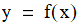A generalized one variable function additionally takes a set of parameters, p, which may appear in the function expression in arbitrary ways: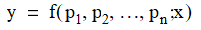For example, this code computes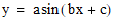:

Code Example – C# nonlinear least squares fit

```public double MyFunction( DoubleVector p, double x )
```
```{
```
```  return p * Math.Sin( p * x + p );
```
```}
```

Code Example – VB nonlinear least squares fit

```Public Function MyFunction(P As DoubleVector, X As Double) As
```
```  Double
```
```  Return P(0) * Math.Sin(P(1) * X + P(2))
```
```End Function
```

Encapsulating One Variable Functions

In NMath, generalized one variable functions can be encapsulated in two ways:

By extending the abstract class , and implementing the Evaluate() method. The GradientWithRespectToParams() can also be implemented to compute the gradient with respect to the parameters; otherwise, a numerical approximation is used.

By wrapping a Func<DoubleVector, double, double> delegate in a . An Action<DoubleVector, double, DoubleVector> delegate can also be provided for computing the gradient with respect to the parameters; otherwise a numerical approximation is used.

For example, this code encapsulates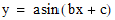using a DoubleParameterizedFunction:

Code Example – C# nonlinear least squares fit

```public class MyFunction : DoubleParameterizedFunction
```
```{
```
```  public MyFunction()
```
```  {}

```

```  public override double Evaluate( DoubleVector p, double x )
```
```  {
```
```    return p * Math.Sin( p * x + p );
```
```  }
```
```}

```

```DoubleParameterizedFunction f = new MyFunction();
```

Code Example – VB nonlinear least squares fit

```Public Class MyFunction
```
```  Inherits DoubleParameterizedFunction

```

```  Public Sub New()
```
```  End Sub

```

```  Public Overrides Function Evaluate(P As DoubleVector,
```
```    X As Double) As Double
```
```    Return P(0) * Math.Sin(P(1) * X + P(2))
```
```  End Function

```

```End Class

```

```Dim F As DoubleParameterizedFunction = New MyFunction()
```

This code encapsulates the same function using a DoubleParameterizedDelegate:

Code Example – C# nonlinear least squares fit

```public double MyFunction( DoubleVector p, double x )
```
```{
```
```  return p * Math.Sin( p * x + p );
```
```}

```

```var f = new DoubleParameterizedDelegate( MyFunction );
```

Code Example – VB nonlinear least squares fit

```Public Function MyFunction(P As DoubleVector, X As Double) As
```
```  Double
```
```  Return P(0) * Math.Sin(P(1) * X + P(2))
```
```End Function

```

```Dim F As New DoubleParameterizedDelegate(AddressOf MyFunction)
```

This code demonstrates implementing GradientWithRespectToParams() as well as Evaluate() in a DoubleParameterizedFunction which encapsulates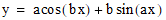:

Code Example – C# nonlinear least squares fit

```public class MyFunction : DoubleParameterizedFunction
```
```{
```
```  public MyFunction()
```
```  {}

```

```  public override double Evaluate( DoubleVector p, double x )
```
```  {
```
```    double a = p;
```
```    double b = p;
```
```    return a*Math.Cos( b*x ) + b*Math.Sin( a*x );
```
```  }

```

```  public override void GradientWithRespectToParams( DoubleVector p,
```
```    double x, ref DoubleVector grad )
```
```  {
```
```    double a = p;
```
```    double b = p;
```
```    grad = Math.Cos( b*x ) + b*x*Math.Cos( a*x );
```
```    grad = -a*x*Math.Sin( b*x ) + Math.Sin( a*x );
```
```  }
```
```}
```

Code Example – VB nonlinear least squares fit

```Public Class MyFunction
```
```  Inherits DoubleParameterizedFunction

```

```  Public Sub New()
```
```  End Sub

```

```  Public Overrides Function Evaluate(P As DoubleVector, X As
```
```    Double) As Double
```
```    Dim A As Double = P(0)
```
```    Dim B As Double = P(1)
```
```    Return a * Math.Cos(b * x) + b * Math.Sin(a * x)
```
```  End Function

```

```  Public Overrides Sub GradientWithRespectToParams(P As
```
```    DoubleVector, X As Double, ByRef Grad As DoubelVector)
```
```    Dim A As Double = P(0)
```
```    Dim B As Double = P(1)
```
```    Grad(0) = Math.Cos(B * X) + B * X * Math.Cos(A * X)
```
```    Grad(1) = -A * X * Math.Sin(B * X) + Math.Sin(A * X)
```
```  End Sub
```
```End Class
```

Predefined Functions

For convenience, class AnalysisFunctions includes a selection of common generalized one variable functions, as shown in Table 23.

 Delegate Function TwoParameterAsymptoticThreeParameterExponential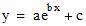ThreeParameterSine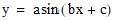FourParameterLogisticFiveParameterLogisticInstances of DoubleParameterizedDelegate can be constructed from these functions. For example:

Code Example – C# nonlinear least squares fit

```var f = new DoubleParameterizedDelegate(
```
```  AnalysisFunctions.FourParameterLogistic );
```

Code Example – VB nonlinear least squares fit

```Dim F As New DoubleParameterizedDelegate(
```
```  AnalysisFunctions.FourParameterLogistic)
```

Constructing a OneVariableFunctionFitter

Class OneVariableFunctionFitter is templatized on INonlinearLeastSqMinimizer, and BoundedOneVariableFunctionFitter is templatized on IBoundedNonlinearLeastSqMinimizer (Section 32.1). Instances are constructed from an encapsulated, generalized one variable function. For example, this code uses one of the predefined curves in AnalysisFunctions:

Code Example – C# nonlinear least squares fit

```var f = new DoubleParameterizedDelegate(
```
```  AnalysisFunctions.FourParameterLogistic );

```

```var fitter =
```
```  new OneVariableFunctionFitter<TrustRegionMinimizer>( f );
```

Code Example – VB nonlinear least squares fit

```Dim F As New DoubleParameterizedDelegate(
```
```  AnalysisFunctions.FourParameterLogistic)

```

```Dim Fitter As New OneVariableFunctionFitter(
```
```  Of TrustRegionMinimizer)(F)
```

As a convenience, there is a constructor that takes a Func<DoubleVector, double, double> delegate directly:

Code Example – C# nonlinear least squares fit

```BoundedOneVariableFunctionFitter<TrustRegionMinimizer> fitter =
```
```  new BoundedOneVariableFunctionFitter<TrustRegionMinimizer>(
```
```    AnalysisFunctions.FourParameterLogistic );
```

Code Example – VB nonlinear least squares fit

```Dim Fitter As New BoundedOneVariableFunctionFitter(
```
```  Of TrustRegionMinimizer)(AnalysisFunctions.FourParameterLogistic)
```

An existing minimizer instance can also be passed to the constructor:

Code Example – C# nonlinear least squares fit

```var minimizer = new LevenbergMarquardtMinimizer();
```
```minimizer.GradientTolerance = 1e-6;

```

```var fitter =
```
```  new OneVariableFunctionFitter<LevenbergMarquardtMinimizer>(
```
```    AnalysisFunctions.FourParameterLogistic, minimizer );
```

Code Example – VB nonlinear least squares fit

```Dim Minimizer As New LevenbergMarquardtMinimizer()
```
```Minimizer.GradientTolerance = "1e-6"

```

```Dim Fitter As New OneVariableFunctionFitter(
```
```  Of LevenbergMarquardtMinimizer)(
```
```    AnalysisFunctions.FourParameterLogistic, Minimizer)
```

Fitting Data

Once you've constructed an instance of OneVariableFunctionFitter or BoundedOneVariableFunctionFitter containing a function, you can fit that function to a set of points using the Fit() method.

The Fit() method on OneVariableFunctionFitter takes vectors of x and y values representing the data points, and a starting position in the function parameter space. For instance:

Code Example – C# nonlinear least squares fit

```var x = new DoubleVector( 0.00, 0.00, 0.00, 0.00, 0.00,
```
```                          0.00, 0.94, 0.94, 0.94, 1.88,
```
```                          1.88, 1.88, 3.75, 3.75, 3.75,
```
```                          7.50, 7.50, 7.50, 15.00, 15.00,
```
```                          15.00, 30.00, 30.00, 30.00 );

```

```var y = new DoubleVector( 7.58, 8.00, 8.32, 7.25, 7.37,
```
```                          7.96, 8.35, 6.91, 7.75, 6.87,
```
```                          6.45, 5.92, 1.92, 2.88, 4.23,
```
```                          1.18, 0.85, 1.05, 0.68, 0.52,
```
```                          0.82, 0.25, 0.22, 0.44 );
```
```
```
```var start = new DoubleVector( "0.1 0.1 0.1 0.1" );

```

```DoubleVector solution = fitter.Fit( x, y, start );
```

Code Example – VB nonlinear least squares fit

```Dim X As New DoubleVector(0.0, 0.0, 0.0, 0.0, 0.0,
```
```                          0.0, 0.94, 0.94, 0.94, 1.88,
```
```                          1.88, 1.88, 3.75, 3.75, 3.75,
```
```                          7.5, 7.5, 7.5, 15.0, 15.0,
```
```                          15.0, 30.0, 30.0, 30.0)

```

```Dim Y As New DoubleVector(7.58, 8.0, 8.32, 7.25, 7.37,
```
```                          7.96, 8.35, 6.91, 7.75, 6.87,
```
```                          6.45, 5.92, 1.92, 2.88, 4.23,
```
```                          1.18, 0.85, 1.05, 0.68, 0.52,
```
```                          0.82, 0.25, 0.22, 0.44)

```

```Dim Start As New DoubleVector("0.1 0.1 0.1 0.1")

```

```Dim Solution As DoubleVector = Fitter.Fit(X, Y, Start)
```

In the space of the function parameters, beginning at a specified start point, Fit() finds a minimum (possibly local) in the sum of the squared residuals with respect to the given x and y values.

NOTE—You must supply at least as many data points to fit as your function has parameters.

The Fit() method on BoundedOneVariableFunctionFitter additionally accepts linear bounds on the solution:

Code Example – C# nonlinear least squares fit

```var lowerBounds = new DoubleVector( 1.1, 1.8 );
```
```var upperBounds = new DoubleVector( 2.1, 3.9 );
```
```DoubleVector solution =
```
```  fitter.Fit( x, y, start, lowerBounds, upperBounds );
```

Code Example – VB nonlinear least squares fit

```Dim LowerBounds As New DoubleVector(1.1, 1.8)
```
```Dim UpperBounds As New DoubleVector(2.1, 3.9)
```
```Dim Solution As DoubleVector = fitter.Fit(X, Y, Start, LowerBounds,
```
```  UpperBounds)
```

Trying different initial starting points is recommended for better solutions. If possible, use starting points based on a priori information about the curve shape and the data being fit. Otherwise, random value close to zero are usually a good choice.

Fit Results

The Fit() method returns the solution found by the minimization. To compute the residuals relative to the data points at the solution, use the ResidualVector() method:

Code Example – C# nonlinear least squares fit

```DoubleVector residuals = fitter.ResidualVector( x, y, solution );
```

Code Example – VB nonlinear least squares fit

```Dim Residuals As DoubleVector =
```
```  fitter.ResidualVector(X, Y, solution)
```

Additional information about the last performed fit is available from the underlying minimizer instance, accessible using the Minimizer property. For example, this code gets the sum of the squared residuals at the starting point and at the solution, the number of iterations performed, and the stop criterion:

Code Example – C# nonlinear least squares fit

```INonlinearLeastSqMinimizer minimizer = fitter.Minimizer;

```

```double initialResidual = minimizer.InitialResidual;
```
```double finalResidual = minimizer.FinalResidual;
```
```int iterations = minimizer.Iterations;
```

Code Example – VB nonlinear least squares fit

```Dim Minimizer As INonlinearLeastSqMinimizer = Fitter.Minimizer

```

```Dim InitialResidual As Double = Minimizer.InitialResidual
```
```Dim FinalResidual As Double = Minimizer.FinalResidual
```
```Dim Iterations As Integer = Minimizer.Iterations
```

NOTE—For testing the goodness of fit of OneVariableFunctionFitter solutions, see class Goodnes­sOfFit. Available statistics include the residual standard error, the coeffi­cient of determination (R2 and "adjusted" R2), the F-statistic for the overall model with its numerator and denominator degrees of freedom, and standard errors, t-statistics, and finally corresponding (two-sided) p-values for the model parameters.

Top

Top